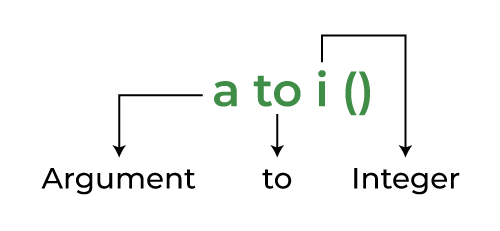GFG App
Open AppBrowser
Continue

# Convert String to int in C

Converting string to int is a reoccurring task in the programming world. Despite being a simple task, many coders either fail or get confused while doing this. Conversion is mostly done so that we can perform operations over numbers that are stored as strings.

Example:

str=”163″

number=163

C is a strongly typed language. We’ll get an error if we try to input a value that isn’t acceptable with the data type. Not just in inputs but we will get an error while performing operations.

There are 3 methods to convert a string to int which are as follows:

1. Using atoi( )
2. Using Loops
3. Using sscanf()

## 1. String Conversion using atoi( )

The atoi() function in C takes a character array or string literal as an argument and returns its value in an integer. It is defined in the <stdlib.h> header file.

If you observe atoi() a little closer you will find out that it stands for:Breakdown of atoi() in simple terms

Example:

## C

 `// C program to demonstrate the ` `// functioning of the atoi() function ` `#include ` `#include ` `int` `main() ` `{ ` `    ``char``* str1 = ``"141"``; ` `    ``char``* str2 = ``"3.14"``; ` ` `  `    ``// explicit type casting ` `    ``int` `res1 = ``atoi``(str1); ` `    ``// explicit type casting ` `    ``int` `res2 = ``atoi``(str2); ` ` `  `    ``printf``(``"atoi(%s) is %d \n"``, str1, res1); ` `    ``printf``(``"atoi(%s) is %d \n"``, str2, res2); ` ` `  `    ``return` `0; ` `}`

Output

```atoi(141) is 141
atoi(3.14) is 3 ```

Atoi behaves a bit differently for string. Let’s check how:

Example:

## C

 `// C Program to implement ` `// Atoi function with char array ` `#include ` `#include ` ` `  `int` `main() ` `{ ` `    ``char``* str1 = ``"Geek 12345"``; ` `    ``char``* str2 = ``"12345 Geek"``; ` ` `  `    ``int` `num1 = ``atoi``(str1); ` `    ``int` `num2 = ``atoi``(str2); ` ` `  `    ``printf``(``"%d is of '%s'\n"``, num1, str1); ` `    ``printf``(``"%d is of '%s'\n"``, num2, str2); ` ` `  `    ``return` `0; ` `}`

Output

```0 is of 'Geek 12345'
12345 is of '12345 Geek'```

Explanation:

• “Geek 12345”  here  ‘Geek’ is the first word so the answer will be : 0 (No number)
• “12345 Geek” here ‘12345’ is the first word so the answer will be: 12345

## 2. Using Loops

We can use loops to convert a string to an integer by traversing each element of the string one by one and comparing the number characters to their ASCII values to get their numeric values and using some mathematics for generating the integer. The below example demonstrates how to do it.

Example:

## C

 `// C Program to convert string ` `// into integer using for loop ` `#include ` `#include ` ` `  `int` `main() ` `{ ` `    ``char``* str = ``"4213"``; ` `    ``int` `num = 0; ` ` `  `    ``// converting string to number ` `    ``for` `(``int` `i = 0; str[i] != ``'\0'``; i++) { ` `        ``num = num * 10 + (str[i] - 48); ` `    ``} ` ` `  `    ``// at this point num contains the converted number ` `    ``printf``(``"%d\n"``, num); ` `    ``return` `0; ` `}`

Output

`4213`

Note: We have used str[i] – 48 to convert the number character to their numeric values. For e.g. ASCII value of character ‘5’ is 53, so 53 – 48 = 5 which is its numeric value.

## 3. Using sscanf()

We can use sscanf() to easily convert a string to an integer. This function reads the formatted input from the string.

Syntax of sscanf:

`int sscanf (const char * source, const char * formatted_string, ...);`

Parameters:

• source  –  source string.
• formatted_string  –  a string that contains the format specifiers.
• … :  –  variable arguments list that contains the address of the variables in which we want to store input data.

There should be at least as many of these arguments as the number of values stored by the format specifiers. On success, the function returns the number of variables filled. In the case of an input failure, before any data could be successfully read, EOF is returned.

Example:

## C

 `// C program to demonstrate ` `// the working of SSCANF() to ` `// convert a string into a number ` `#include ` ` `  `int` `main() ` `{ ` `    ``const` `char``* str1 = ``"12345"``; ` `    ``const` `char``* str2 = ``"12345.54"``; ` `    ``int` `x; ` ` `  `    ``// taking integer value using %d format specifier for ` `    ``// int ` `    ``sscanf``(str1, ``"%d"``, &x); ` `    ``printf``(``"The value of x : %d\n"``, x); ` ` `  `    ``float` `y; ` `    ``// taking float value using %f format specifier for ` `    ``// float ` `    ``sscanf``(str2, ``"%f"``, &y); ` `    ``printf``(``"The value of x : %f\n"``, y); ` ` `  `    ``return` `0; ` `}`

Output

```The value of x : 12345
The value of x : 12345.540039```

### Can we typecast String to int?

The answer is NO. If we use typecasting to convert the string to a number, we will get an error as shown in the example below.

Example:

## C

 `// C Program to check  the output ` `// of typecasting from string to integer ` `#include ` ` `  `int` `main() ` `{ ` `    ``string str = ``"8"``; ` `    ``int` `num; ` ` `  `      ``// Typecasting  ` `    ``num = (``int``)str; ` `    ``return` `0; ` `}`

Output:

```main.c: In function ‘main’:
main.c:9:11: warning: cast from pointer to integer of different size [-Wpointer-to-int-cast]
9 |     num = (int)str;
|           ^
1683652612```

Explanation: As both string and int are not in the same object hierarchy, we cannot perform implicit or explicit type casting as we can do in case of double to int or float to int conversion.

In the above code, we can see the output gives the warning with any garbage value inside it. So, to avoid such conditions, we use the methods specified above.

My Personal Notes arrow_drop_up